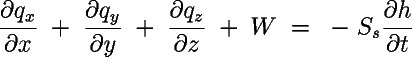# EPA On-line Tools for Site Assessment Calculation

 47 of 45

#### Ground Water Flow

The three dimensional ground water flow equation is given bywhere qx is the Darcy flux, h is the hydraulic head, Kx is the hydraulic conductivity in the x direction, and Ss is the storage coefficient for a confined aquifer. The Darcy flux is defined bywhere qx is the Darcy flux in the x direction and i is the hydraulic gradient.Three calculators address components of Darcy's law: Gradient Calculators Hydraulic Conductivity Unit Conversions Darcy's Law The subsequent pages show examples for the use of these calculators.

 Previous Top ^ Next

Home | Glossary | Notation | Links | References | Calculators

Contact Jim Weaver to ask a technical question on this material.